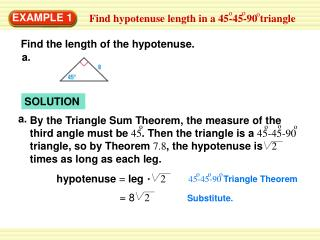DownloadDownload PresentationEXAMPLE 1

# EXAMPLE 1

Download Presentation## EXAMPLE 1

- - - - - - - - - - - - - - - - - - - - - - - - - - - E N D - - - - - - - - - - - - - - - - - - - - - - - - - - -
##### Presentation Transcript

1. o o o Find hypotenuse length in a 45-45-90triangle a. a. By the Triangle Sum Theorem, the measure of the third angle must be 45. Then the triangle is a 45-45-90 triangle, so by Theorem 7.8, the hypotenuse is 2 times as long as each leg. o o o o o o o hypotenuse = leg 2 45-45-90 Triangle Theorem = 8 2 EXAMPLE 1 Find the length of the hypotenuse. SOLUTION Substitute.

2. o o o Find hypotenuse length in a 45-45-90triangle b. o o o hypotenuse = leg 2 45-45-90 Triangle Theorem By the Base Angles Theorem and the Corollary to the Triangle Sum Theorem, the triangle is a triangle. b. = 3 2 2 = 3 2 o o o 45 - 45 - 90 EXAMPLE 1 Find the length of the hypotenuse. Substitute. Product of square roots = 6 Simplify.

3. o o o Find leg lengths in a 45-45-90triangle By the Base Angles Theorem and the Corollary to the Triangle Sum Theorem, the triangle is a triangle. o o o hypotenuse = leg 2 45-45-90 Triangle Theorem = x 5 2 2 2 2 2 2 o o o 45 - 45 - 90 x 5 = Divide each side by 2 EXAMPLE 2 Find the lengths of the legs in the triangle. SOLUTION Substitute. 5 =x Simplify.

4. By the Corollary to the Triangle Sum Theorem, the triangle is a triangle. o o o 45 - 45 - 90 EXAMPLE 3 Standardized Test Practice SOLUTION

5. hypotenuse = leg WX = 25 The correct answer is B. 2 2 EXAMPLE 3 Standardized Test Practice o o o 45-45-90 Triangle Theorem Substitute.

6. By the Base Angles Theorem and the Corollary to the Triangle Sum Theorem, the triangle is a triangle. o o o hypotenuse = leg 45-45-90 Triangle Theorem 2 x = 2 2 2 2 2 2 2 o o o 45 - 45 - 90 x 2 = Divide each side by 2 for Examples 1,2 and 3 GUIDED PRACTICE Find the value of the variable. 1. SOLUTION Substitute. 2 = x Simplify.

7. By the Base Angles Theorem and the Corollary to the Triangle Sum Theorem, the triangle is a triangle. o o o hypotenuse = leg 45-45-90 Triangle Theorem 2 2 y = 2 o o o 45 - 45 - 90 for Examples 1,2 and 3 GUIDED PRACTICE Find the value of the variable. 2. SOLUTION Substitute. y = 2 Simplify.

8. By the Base Angles Theorem and the Corollary to the Triangle Sum Theorem, the triangle is a triangle. o o o hypotenuse = leg 45-45-90 Triangle Theorem 2 2 d = 8 2 d = 8 o o o 45 - 45 - 90 for Examples 1,2 and 3 GUIDED PRACTICE Find the value of the variable. 3. SOLUTION Substitute. Simplify.

9. By the Base Angles Theorem and the Corollary to the Triangle Sum Theorem, the triangle is a triangle. o o o hypotenuse = leg 45-45-90 Triangle Theorem 2 2 2 2 2 2 6 = leg 3 = leg o o o Divide each side by 2 45 - 45 - 90 3 = leg for Examples 1,2 and 3 GUIDED PRACTICE 4. Find the leg length of a 45°-45°-90° triangle with a hypotenuse length of 6. SOLUTION Substitute. Simplify.